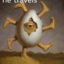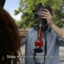MathematicsOpenStudy (anonymous):

The graph of function y=x^3+6x^2+7x-2cos x, changes concavity at x=? Could someone please help me with this math problem, I'm having a hard time understanding how to get the correct answer.myininaya (myininaya):

$y'=3x^2+12x+7+2\sin(x) =>y''=6x+12+0+2\cos(x)$ $y''=0=>6x+12+2\cos(x)=0=>6x+2\cos(x)=-12$myininaya (myininaya):

i suggest using a graphing tool to find where the concavity changes. solving 6x+2cos(x)=-12 is not possible i believemyininaya (myininaya):

solving it algebraically i meanOpenStudy (anonymous):

Could you explain how to solve it with a graphing calculator. I have one that I can use, it's just I'm not sure what steps to use on the calculator to go about solving it. >_<myininaya (myininaya):

do you know how to find the derivative?OpenStudy (anonymous):

yes i found the 1st and 2nd derivative i'm just not sure what to do after that.myininaya (myininaya):myininaya (myininaya):

where your graph intersects the x-axis is where you have inflection pointsOpenStudy (anonymous):

and the inflection points are where the concavity changes, right?OpenStudy (anonymous):

and the inflection points are where the concavity changes, right?OpenStudy (anonymous):

okay i got it now thanks!myininaya (myininaya):

yes rightOpenStudy (anonymous):

Thank you again for all the help, I very much appreciated it!!

Latest QuestionsShelbiLW: A 3.0 m uniform beam of mass 15 kg is pivoted 1.0 m from the end as shown below. A 35 kg child sits 0.
4 hours ago 0 Replies 0 Medalsryleighmullins22: Answer the question below using the following amortization table. Month Principal Paid Interest Paid Balance 21 \$654.
5 hours ago 0 Replies 0 MedalsTonycoolkid21: help
5 hours ago 8 Replies 0 MedalsTonycoolkid21: help
5 hours ago 15 Replies 3 MedalsRx1nyDxyz: Help If the supply of a product increases, then the price decreases*** the price
6 hours ago 21 Replies 1 Medalshototodorokii: (not from a test/quiz)Nomenclature is governed by certain universal rules. Which
7 hours ago 0 Replies 0 Medalscrispyrat: A guide to sqrt and cbrt
5 hours ago 21 Replies 3 MedalsxXQuintonXx: help please(ss below)
7 hours ago 5 Replies 1 Medalajfknkjfa: Prove that the two circles shown below are similar. (10 points) Circle C is shown with a center at negative 3, 1 and a radius of 4.
7 hours ago 3 Replies 1 Medalrxcklesskaisher: idk wdh dis is
7 hours ago 2 Replies 0 Medals